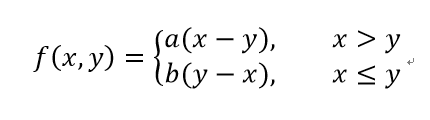# TensorFlow自定义损失函数

TensorFlow提供了许多的损失函数，在训练模型中经常使用的损失函数有交叉熵、平方差。TensorFlow除了提供这些损失函数之外，还给开发者提供了一种自定义损失函数的方法。让开发者，可以根据实际项目的需要来自定义损失函数，让我们可以更好的训练出满足开发者需要的模型。1、tf.greater(x,y,name=None)函数介绍

x：是一个张量（Tensor），这个张量必须是float32、float64、int32，int64、uint8、int16、int8、uint16、half类型。

y：是一个张量（Tensor），这个张量的类型必须和x一样。

name：操作的名称（可选）。

import tensorflow as tf

if __name__ == "__main__":
#定义一个张量a
a = tf.constant([1.,2.])
#定义一个张量b
b = tf.constant([3.,4.])
#创建一个session并设置为默认session
sess = tf.InteractiveSession()
#比较a和b的大小，如果前一个大于后一个为True，否则为False
print(tf.greater(a,b).eval())
# [False False]
print(tf.greater(b,a).eval())
#[ True  True]

import tensorflow as tf

if __name__ == "__main__":
#定义一个张量a
a = tf.constant([1.,4.])
#定义一个张量b
b = tf.constant([3.])
#创建一个session并设置为默认session
sess = tf.InteractiveSession()
#比较a和b的大小，如果前一个大于后一个为True，否则为False
print(tf.greater(a,b).eval())#实际比较的时候，类似于将张量b扩展成了[3.,3.]之后，再与a进行比较
# [False  True]
print(tf.greater(b,a).eval())
#[ True False]
2、tf.where(condition,x=None,y=None,name=None)

condition：一个张量元素的类型为bool。

x：是一个张量与张量condition有同样的大小，如果condition是1阶的，x可以有更高阶，但是第一个维度的大小必须和conditio一致。

y：是一个与x有同样大小的张量

name：操作的名称（可选）。

import tensorflow as tf

if __name__ == "__main__":
#定义一个张量a
a = tf.constant([1.,2.,3.])
#定义一个张量b
b = tf.constant([4.,5.,6.])
#创建一个session并设置为默认session
sess = tf.InteractiveSession()
print(tf.where([True,False,True],a,b).eval())
# [ 1.  5.  3.]

import tensorflow as tf

if __name__ == "__main__":
#定义一个张量a
a = tf.constant([[1.,2.,3.],[1,2,3]])
#定义一个张量b
b = tf.constant([[4.,5.,6.],[7,8,9]])
#创建一个session并设置为默认session
sess = tf.InteractiveSession()
print(tf.where([True,False],a,b).eval())
'''
[[ 1.  2.  3.]
[ 7.  8.  9.]]
'''
print(tf.where([[True, False,True],[True, False,True]], a, b).eval())
'''
[[ 1.  5.  3.]
[ 1.  8.  3.]]
'''

import tensorflow as tf
from numpy.random import RandomState

if __name__ == "__main__":
#设置每次迭代时数据的大小
batch_size = 8
#定义输入和输出节点
#定义输入节点，设置输入的数据为1行2两列，设置None的目的是表示不确定输入数据的个数
#最后，我们会将整个的数据数据整合成一个矩阵
x = tf.placeholder(tf.float32,shape=(None,2),name='x-input')
#定义输出节点，保证每个输入值所对应的输出值为1维的
y_ = tf.placeholder(tf.float32,shape=(None,1),name='y-input')
#定义神经网络的前向传播过程
#定义参数，设置参数的大小为两行一列
w1 = tf.Variable(tf.random_normal([2,1],stddev=1,seed=1))
#输入矩阵与参数进行加权和
y = tf.matmul(x,w1)
#定义预测多了和预测少了的损失的利润
loss_less = 5
loss_more = 1
#定义损失函数
loss = tf.reduce_sum(tf.where(tf.greater(y,y_),(y-y_)*loss_more,(y_-y)*loss_less))
#最小化损失函数
#随机生成一个数据集
rdm = RandomState(1)
#设置数据集的大小
dataset_size = 128
#产生输入数据
X = rdm.rand(dataset_size,2)
#定义输出y
'''
设置样本的输出值为，两个输入值相加并加上一个随机量。
还为输出值设置了一个-0.05~0.05的随机数噪音，达到模拟真实数据的效果。
'''
Y = [[x1+x2 + rdm.rand()/5.0 - 0.05] for (x1,x2) in X]
#训练神经网络
with tf.Session() as sess:
#初始化参数变量
init_op = tf.initialize_all_variables()
sess.run(init_op)
#设置迭代次数
STEPS = 5000
for i in range(STEPS):
start = (i * batch_size) % dataset_size
end = min(start + batch_size,dataset_size)
#训练模型
sess.run(train_step,feed_dict={x:X[start:end],y_:Y[start:end]})
print(w1.eval())
#[[ 1.08637631]
# [ 1.12113917]]06-142万+
06-142万+
04-14456
11-121613
02-034181
02-144858
07-023012
06-134260
08-241万+点击重新获取扫码支付余额充值